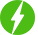# Reasoning- Matrices Number

2

Reasoning- Matrices Number, Matrices number.

## Course Description

Welcome to the reasoning topic matrix number in this you will get solve different type of matrices

b) Subtraction in Matrices

c) Cube in Matrices

d) Square in Matrices

e) Sum of All in Matrices

f) Division of Matrices

Their 10 Question solved on Matrices

Question Number 01- in this question you are going to see  multiple of numbers

Question Number 02 – in this question you are going to see  square adding of numbers

Question Number 03 – in this question you are going to see  subtraction and multiple of numbers

Question Number 04 – in this question you are going to see  additional and division of numbers

Question Number 05 – in this question you are going to see  multiple and division of numbers

Question Number 06 – in this question you are going to see adding in numbers

Question Number 07 – in this question you are going to see subtraction and cube of numbers

Question Number 08 – in this question you are going to see  squaring and subtraction of numbers

Question Number 09 – in this question you are going to see  sum of all of numbers

Question Number 10 – in this question you are going to see squaring of numbers

you will get better understand how to solve this type of matrices problem in competitive exam also in reasoning part in interview. I hope that you will easily understand video and try to solve question. you can easily solve matrices in future by watching this content which is made for you all. I hope that you will get this video and you will try to solve quiz season also. thanks for taking this course

We will be happy to hear your thoughtsRegister New Account
• Total (0)
0